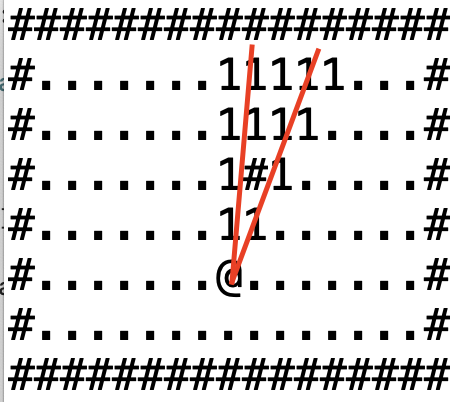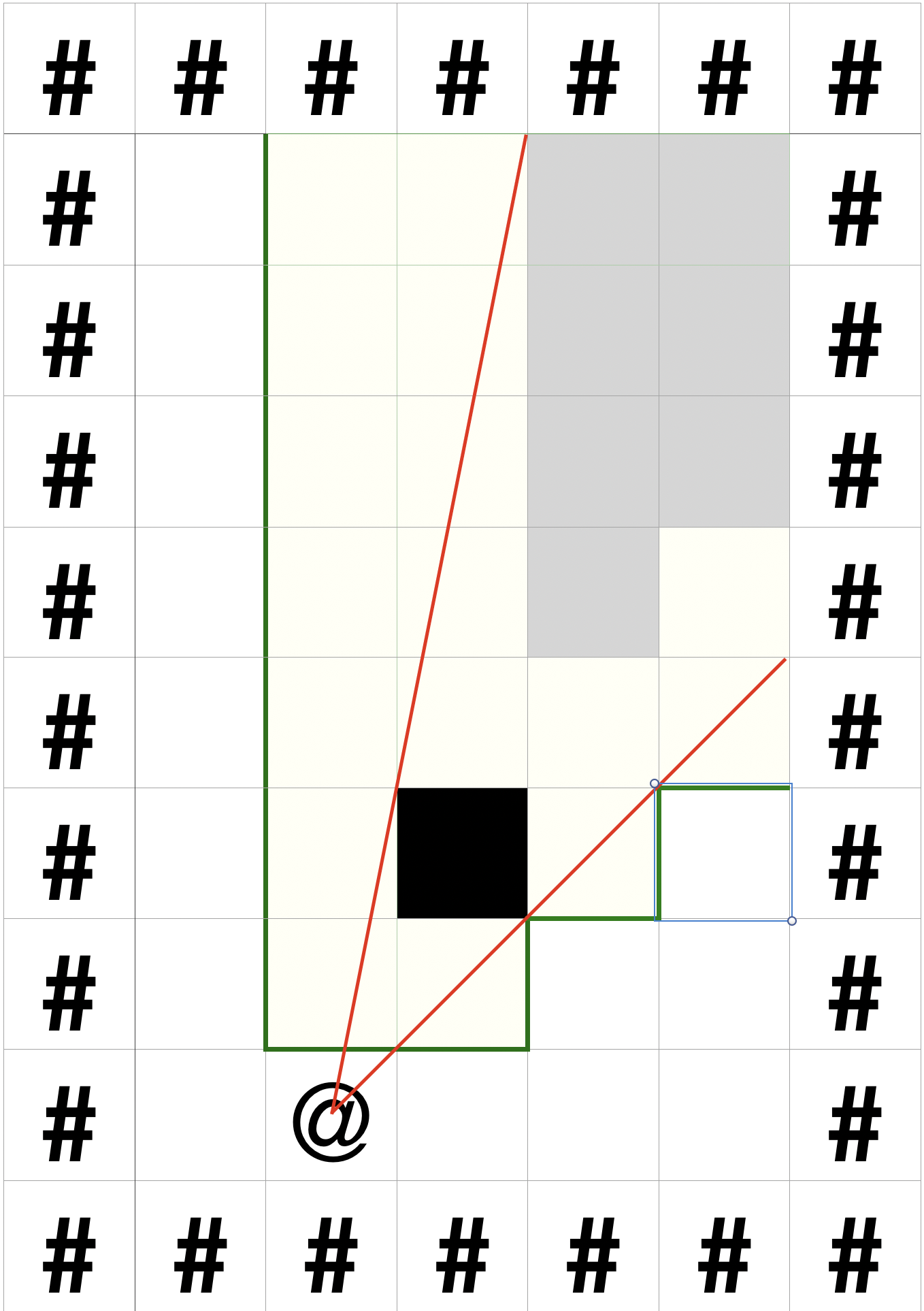.O.
..O Deniz's personal pages
OOO

## Roguelike FOV 2

July 18, 2020

I was thinking that the ray casting method above would be sufficient but I have since seen some quirks in the highlighted tiles in the FoV that made me want to ditch it. Another reason for implementing a more efficient algorithm is that the enemies and neutral characters in the level also have a field of view that will make them react to other actors, props, etc. that they can see. This meant that the FoV calculations will be triggered over and over for potentially tens to hundreds of actors per turn.

Shadow casting is actually a reversal in a sense that you don’t try to figure out the cells that are visible but try to find the cells that are shadowed by obstacles. After some research on previous implementations I was able to grasp the logic behind the algorithm and want to go over it here with hopes to explain it better.

Ultimately we want to have a 360 degree FoV but breaking up the whole into 8 pieces of 45 degrees each will make it much more simpler as the algorithm is basically the same for each octant with just the increments of the rows and columns differing by octant.

#################f
#.......11111...#e
#.......1111....#d
#.......111.....#c
#.......11......#b
#.......@.......#a
#...............#
#################


the first octant marked by 1’s

we will scan each row in the octant and mark it as visible if there is no object that is cast a shadow, starting for row a working out way up to row f. You should terminate processing if the FoV radius is reached before the end of the map. So without any blocking tiles this is rather straight forward.

for row in 1..<actor.fovRadius {
for col in 0...row {
y = player.position.y - row
x = player.position.x + col
map[y][x] = 1
}
}


Then we need to repeat the same for the remaining 7 octants. The part that will change is the player.position.y - row and player.position.x + col. For the octant that covers [-45;0] you would want to subtract the column instead of adding it. A nice way of implementing this would be to store the increment deltas in a list and apply them based on the octant number

let octants = [
[XY(x: 0, y: -1), XY(x: 1, y: 0)],
[XY(x: 1, y: 0), XY(x: 0, y: -1)],
[XY(x: 1, y: 0), XY(x: 0, y: 1)],
[XY(x: 0, y: 1), XY(x: 1, y: 0)],
[XY(x: 0, y: 1), XY(x: -1, y: 0)],
[XY(x: -1, y: 0), XY(x: 0, y: 1)],
[XY(x: -1, y: 0), XY(x: 0, y: -1)],
[XY(x: 0, y: -1), XY(x: -1, y: 0)]
]


Now the increments can be accessed as

let rowInc = octants[octant]
let colInc = octants[octant]
and we can iterate over this list for each octant we want to process
let fov = (0..<8).flatMap { processOctant(actor: actor, octant: $0) } with the private func processOctant(actor: Character, octant: Int) -> [XY] { ... }  Ok, so how can we calculate the shadows? The idea is to keep a list of shadow angles that are being cast from an opaque object. We’ll define the angle to be a value between 0 and 1 where 0 represents 0 degrees and 1 represents 45 degrees in the first octant. This will have different values for the other octants but that’s not important. We’re not really dealing with the angles but the slope of the ray that touches from the top left of the obstacle and the bottom right proceeding upwards and right (for the 1st octant) that is originating from the player.Here’s a zoomed in versionlight rays shooting from the player Any tile that has a projection (lights shooting from the player touch the top left and bottom right) that falls within the range of already calculated projections cannot be seen by the player. This is due to the fact that any tile further away from the player will have a narrower angle between the left and right slopes because all of the tiles are the same size and shape. Calculating the slopes for the projection is basically finding the ratio of the columns to rows of the top left and bottom right parts of the tile. By rows and columns I mean the length of the line from the player tile to the target tile. private func getProjection(row: Int, col: Int) -> Shadow { let topLeft = Double(col) / Double(row + 1) let bottomRight = Double(col + 1) / Double(row) return Shadow(start: topLeft, end: bottomRight) }  for the lop left calculation the nominator is col as the player location is zero so target location - player location is just the column of the target. The denominator has 1 added to its row because the it’s actually the bottom of the row above it. For the bottom right calculation we need to add 1 to the column as it’s actually the corner of the next column. As we are processing row by row we need to keep track of all the shadows (left and right slopes) to filter out tiles that fall into this range. We could keep a list of all the left/right slopes we have seen thus far and linearly search each one but there is a better way: we can actually merge any new projections that we encounter into a list of existing projections. This works like this: Let’s say our shadow list is [(0…0.2), (0.6…0.7)] and we get the projection (0.8…0.9). We check out list and see that it doesn’t intersect with any existing projections so we can just append it to our list. Out new list is [(0…0.2), (0.6…0.7), (0.8…0.9)]. Lets say our next tile has the projection [0.4…0.85]. This fits right into the middle of and covers the existing (0.6…0.7) projection entirely and also partially covers (0.8…0.9) so we can go ahead and merge. Our new list is now [(0…0.2), (0.4…0.9)] Instead of having 4 items we have 2 now that we merged. If we ever reach a state where we have only one element in the shadows list and the left slope is 0 and right slope is 1 then we have the whole octant covered and every object in the rows after this row will be covered by the shadow so we can stop processing. private func processOctant(actor: Character, octant: Int) -> [XY] { let rowInc = octants[octant] let colInc = octants[octant] var fullShadow = false var result = [XY]() shadows = [Shadow]() for row in 1..<actor.fovRadius { var pos = actor.location + (rowInc * row) guard actor.game.scene.viewPort.contains(point: pos) else { break } for col in 0...row { if fullShadow { continue } else { let projection = getProjection(row: row, col: col) if !isInShadow(projection: projection) { result.append(pos) } if actor.game.level.map[pos.y][pos.x].blocking { fullShadow = addShadow(shadow: projection) } } pos = pos + colInc guard actor.game.scene.viewPort.contains(point: pos) else { break } } } return result }  So how would this merging algorithm look like? Here are the steps we need to consider 1. Find out the correct index to put our new item in. It could be with or without a merge. 2. Find if our new item overlaps with the previous entry or the next entry. We’ll use this to do any necessary merges. 3. handle the 4 conditions of 1. overlaps with previous and next 2. overlaps with next but not previous 3. overlaps with previous but not next 4. there is no overlap at all 5. based on the overlapping situation adjusting the start/end (left/right) slope will take care of the merging. var index = 0 for curShadow in shadows { if curShadow.start > shadow.start { break } index += 1 } //let index = shadows.firstIndex {$0.start > shadow.start } ?? shadows.count

let overlapsPrev = (index > 0) && (shadows[index - 1].end > shadow.start)

if overlapsNext {
if overlapsPrev {